# JP6115781B2 - Image processing apparatus and image processing method - Google Patents

## Info

Publication number
JP6115781B2
JP6115781B2 JP2013528428A JP2013528428A JP6115781B2 JP 6115781 B2 JP6115781 B2 JP 6115781B2 JP 2013528428 A JP2013528428 A JP 2013528428A JP 2013528428 A JP2013528428 A JP 2013528428A JP 6115781 B2 JP6115781 B2 JP 6115781B2
Authority
JP
Japan
Prior art keywords
depth
plurality
reliability
image processing
value
Prior art date
Legal status (The legal status is an assumption and is not a legal conclusion. Google has not performed a legal analysis and makes no representation as to the accuracy of the status listed.)
Active
Application number
JP2013528428A
Other languages
Japanese (ja)
Other versions
JPWO2013145554A1 (en
Inventor
グエン カン
グエン カン

Original Assignee
パナソニックＩｐマネジメント株式会社
Priority date (The priority date is an assumption and is not a legal conclusion. Google has not performed a legal analysis and makes no representation as to the accuracy of the date listed.)
Filing date
Publication date
Priority to JP2012078066 priority Critical
Priority to JP2012078066 priority
Application filed by パナソニックＩｐマネジメント株式会社 filed Critical パナソニックＩｐマネジメント株式会社
Priority to PCT/JP2013/001216 priority patent/WO2013145554A1/en
Publication of JPWO2013145554A1 publication Critical patent/JPWO2013145554A1/en
Application granted granted Critical
Publication of JP6115781B2 publication Critical patent/JP6115781B2/en
Application status is Active legal-status Critical
Anticipated expiration legal-status Critical

## Images

•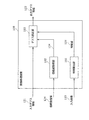•••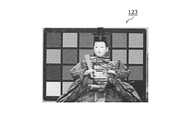•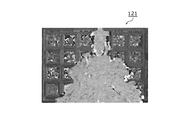•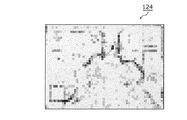•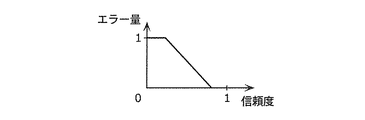•••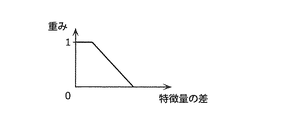•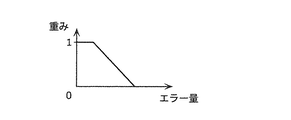•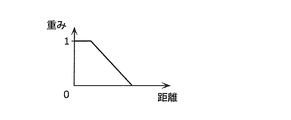•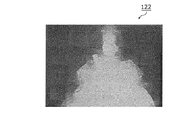•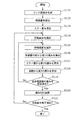•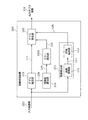•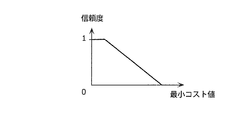•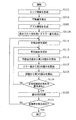••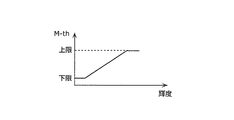•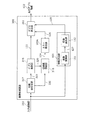•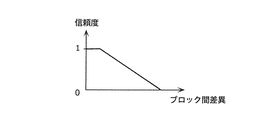•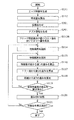## Classifications

• GPHYSICS
• G06COMPUTING; CALCULATING; COUNTING
• G06TIMAGE DATA PROCESSING OR GENERATION, IN GENERAL
• G06T19/00Manipulating 3D models or images for computer graphics
• G06T19/20Editing of 3D images, e.g. changing shapes or colours, aligning objects or positioning parts
• GPHYSICS
• G06COMPUTING; CALCULATING; COUNTING
• G06KRECOGNITION OF DATA; PRESENTATION OF DATA; RECORD CARRIERS; HANDLING RECORD CARRIERS
• G06K9/00Methods or arrangements for reading or recognising printed or written characters or for recognising patterns, e.g. fingerprints
• G06K9/36Image preprocessing, i.e. processing the image information without deciding about the identity of the image
• G06K9/46Extraction of features or characteristics of the image
• GPHYSICS
• G06COMPUTING; CALCULATING; COUNTING
• G06TIMAGE DATA PROCESSING OR GENERATION, IN GENERAL
• G06T5/00Image enhancement or restoration
• G06T5/001Image restoration
• GPHYSICS
• G06COMPUTING; CALCULATING; COUNTING
• G06TIMAGE DATA PROCESSING OR GENERATION, IN GENERAL
• G06T5/00Image enhancement or restoration
• G06T5/001Image restoration
• G06T5/002Denoising; Smoothing
• HELECTRICITY
• H04ELECTRIC COMMUNICATION TECHNIQUE
• H04NPICTORIAL COMMUNICATION, e.g. TELEVISION
• H04N13/00Stereoscopic video systems; Multi-view video systems; Details thereof
• H04N13/10Processing, recording or transmission of stereoscopic or multi-view image signals
• H04N13/106Processing image signals
• H04N13/111Transformation of image signals corresponding to virtual viewpoints, e.g. spatial image interpolation
• GPHYSICS
• G06COMPUTING; CALCULATING; COUNTING
• G06TIMAGE DATA PROCESSING OR GENERATION, IN GENERAL
• G06T2207/00Indexing scheme for image analysis or image enhancement
• G06T2207/10Image acquisition modality
• G06T2207/10028Range image; Depth image; 3D point clouds

## Description

The present invention relates to an image processing apparatus and an image processing method for correcting a depth value.

2. Description of the Related Art Conventionally, a technique for estimating a depth value of a pixel or a fixed area for each pixel or a fixed area included in an input image is known. The depth value is, for example, a distance from the imaging device to the subject, and is also called a depth value. However, in processing using these techniques, detection accuracy may be reduced due to an error in depth estimation due to the influence of image characteristics or noise. For this reason, in the image processing using the depth value generated by the conventional depth estimation technique, the image quality of the output image is degraded. For example, in DIBR (Depth Image Based Rendering) processing that generates a parallax image by shifting an image based on a depth value, there is a problem that noise or object distortion occurs in the generated image due to an error in the depth value. There is. In addition, in the process of arbitrarily adjusting the blur of the image using the depth value, the context of the subject is broken due to a depth value error. As a result, there is a problem that the blur of the subject in the generated image becomes unnatural.

Thus, in order to improve the quality of the output image generated by the image processing using the depth value, a highly accurate depth value is required. However, as described above, there is a limit to the accuracy of the depth value estimation process using an image, and the depth value after estimation includes many errors. Therefore, a technique for correcting the depth value after estimation has been developed.

As a conventional technique for correcting a depth value, there is a technique for correcting a depth value by using a relationship between a pixel value of a target pixel and a pixel value of a peripheral pixel (see, for example, Patent Document 1). The device described in Patent Document 1 calculates a weight of a peripheral depth value using a pixel value (color, luminance, or color difference) of an input image, and corrects the depth value using the weight. In other words, the apparatus corrects the depth value of the pixel by regarding the pixels having similar pixel values as the same subject.

International Publication No. 2011/033673

In such an image processing apparatus that corrects the depth value, it is desired to improve the accuracy of the depth value.

SUMMARY An advantage of some aspects of the invention is that it provides an image processing apparatus capable of improving the accuracy of a depth value.

In order to achieve the above object, an image processing apparatus according to an aspect of the present invention is an image processing apparatus that corrects a plurality of depth values indicating the depths of a plurality of pixels included in an input image. A feature calculation unit that calculates the feature amount of each of the pixels, a reliability acquisition unit that acquires the reliability of each of the plurality of depth values, and the plurality of the plurality of reliability values using the plurality of reliability and the plurality of feature amounts. And a depth correction unit that corrects the depth value.

These general or specific aspects may be realized by a system, a method, an integrated circuit, a computer program, or a recording medium such as a computer-readable CD-ROM. The system, method, integrated circuit, computer program Also, any combination of recording media may be realized.

The present invention can provide an image processing apparatus capable of improving the accuracy of the depth value.

FIG. 1 is a block diagram illustrating a configuration of the image processing apparatus according to the first embodiment. FIG. 2 is a flowchart of the image processing method according to the first embodiment. FIG. 3 is a block diagram illustrating a configuration of the image processing apparatus according to the first embodiment. FIG. 4 is a diagram illustrating an example of an input image according to the first embodiment. FIG. 5 is a diagram illustrating an example of input depth information according to the first embodiment. FIG. 6 is a diagram illustrating an example of reliability information according to the first embodiment. FIG. 7 is a diagram showing a relationship between the reliability and the error amount according to the first embodiment. FIG. 8 is a diagram illustrating an example of an edge image according to the first embodiment. FIG. 9 is a block diagram illustrating a configuration of the depth correction unit according to the first embodiment. FIG. 10A is a diagram showing a relationship between a feature amount difference and a weight according to the first embodiment. FIG. 10B is a diagram showing a relationship between the error amount and the weight according to the first embodiment. FIG. 10C is a diagram showing a relationship between the distance and the weight according to Embodiment 1. FIG. 11 is a diagram illustrating an example of output depth information according to the first embodiment. FIG. 12 is a flowchart of the image processing method according to the first embodiment. FIG. 13 is a block diagram illustrating a configuration of the image processing apparatus according to the second embodiment. FIG. 14 is a diagram illustrating a relationship between the minimum cost value and the reliability according to the second embodiment. FIG. 15 is a flowchart of the image processing method according to the second embodiment. FIG. 16 is a diagram illustrating a relationship between the minimum cost value and the reliability according to the second embodiment. FIG. 17 is a diagram illustrating the relationship between the luminance and the threshold according to the second embodiment. FIG. 18 is a block diagram illustrating a configuration of the image processing apparatus according to the third embodiment. FIG. 19 is a diagram illustrating the relationship between the difference between blocks and the reliability according to the third embodiment. FIG. 20 is a flowchart of the image processing method according to the third embodiment.

(Knowledge that became the basis of the present invention)
The present inventor has found that the following problems occur in the prior art.

For example, the conventional apparatus described in Japanese Patent Laid-Open No. 2004-228561 considers subjects having different colors as the same subject, and may use an incorrect peripheral depth value when correcting the depth value. As a result, the inventor has found that the accuracy of the corrected depth value is reduced.

In addition, a natural image includes many portions with different colors in the same subject. In such a case, the conventional apparatus determines that the portions with different colors are different subjects despite the same subject. As a result, even when the peripheral depth value is correct, the depth value is not used for correction, and thus the depth value of the target pixel is not corrected. As a result, the present inventor has found that the conventional process cannot correct the depth value correctly, and the accuracy of the depth value is not improved.

In order to solve such a problem, an image processing apparatus according to an aspect of the present invention is an image processing apparatus that corrects a plurality of depth values indicating depths of a plurality of pixels included in an input image, and Using the feature calculation unit that calculates the feature amount of each of the plurality of pixels, the reliability acquisition unit that acquires the reliability of each of the plurality of depth values, and the plurality of reliability and the plurality of feature amounts A depth correction unit that corrects the plurality of depth values.

As a result, the image processing apparatus can determine the correctness of the depth value of the pixel to be processed and the depth value of the surrounding image by using the reliability. The depth value of the pixels can be corrected. In addition, pixels having the same characteristics are likely to have the same subject, that is, the same depth value. Therefore, the image processing apparatus can determine, for example, peripheral pixels included in the same subject as the pixel to be processed using the feature amount of the pixel. Thereby, the image processing apparatus can improve the accuracy of the depth value.

For example, the depth correction unit may refer to a feature value that is close to the depth value of the high reliability of the plurality of depth values of the plurality of reference pixels included in the plurality of pixels and the depth value of the target pixel to be processed. The depth value of the target pixel may be corrected using the depth value of the pixel with priority.

Accordingly, the image processing apparatus can correct the depth value of the target pixel using the depth value with high reliability. Furthermore, the image processing apparatus can determine peripheral pixels included in the same subject as the target pixel. Thereby, the image processing apparatus can improve the accuracy of the depth value.

For example, the depth correction unit uses a weight calculation unit that calculates a weight of each reference pixel by using the feature amount and the reliability of each of the plurality of reference pixels, and uses the plurality of calculated weights. A depth synthesis unit that generates the depth value of the target pixel after correction by performing weighted averaging of the depth values of the plurality of reference pixels.

Thereby, the image processing apparatus calculates a weight based on the reliability of the reference pixel. That is, since the weight varies depending on the degree of correctness of the depth value of the reference pixel, the depth value correction accuracy is improved. Further, the image processing apparatus calculates a weight based on the feature amount of the pixel. As a result, the image processing apparatus can use the depth value of a pixel having characteristics similar to that of the processing pixel, for example, so that the accuracy of the depth value can be improved.

For example, the weight calculation unit calculates a difference between the feature amount of the target pixel and each of the feature amounts of the plurality of reference pixels, and the weight of the corresponding reference pixel is decreased as the difference is larger. Also good.

As a result, the weight value increases as the depth value of the reference pixel is similar in characteristics to the target pixel. Therefore, since the image processing apparatus can use many depth values of pixels having similar characteristics, the accuracy of the depth value of the target pixel can be improved.

For example, the feature amount may include edge strength in each pixel.

Accordingly, the image processing apparatus can calculate the feature amounts of the target pixel and the reference pixel. In general, an edge is detected at the boundary between subjects. In addition, the depth value is likely to be different for different subjects. Therefore, the image processing apparatus can determine the reference pixel included in the same subject as the target pixel by using the amount of the edge, and can use the depth value of the reference pixel. Thus, the image processing apparatus can improve the accuracy of the depth value.

For example, the feature amount may include an edge direction in each pixel.

Thereby, the image processing apparatus can correct the depth value using the direction of the edge of the pixel. Thereby, the image processing apparatus can improve the accuracy of the depth value.

For example, the weight calculation unit may increase the weight of the reference pixel as the reliability of the reference pixel increases.

Thus, the image processing apparatus can reduce the influence of pixels including errors by reducing the weight for the depth value with low reliability. Further, the image processing apparatus can improve the accuracy of the corrected depth value by increasing the weight for the depth value with high reliability.

For example, the weight calculation unit may decrease the weight of the reference pixel as the distance between the target pixel and the reference pixel increases.

Here, the pixel farther from the target pixel is more likely to be included in a subject different from the target pixel. That is, the pixel farther away from the target pixel is more likely to have a different depth value from the target pixel. Therefore, the image processing apparatus can reduce the influence of the depth value of a different subject by reducing the weight of the depth value of the pixel far from the target pixel.

For example, the reliability may indicate a degree of correctness of the depth value or whether the depth value is correct.

Thereby, since the image processing apparatus can grasp the correct degree of the depth value, for example, when correcting the depth value of the target pixel, for example, many peripheral correct depth values are distributed to the depth value of the target pixel. It is possible to distribute the incorrect depth value of less. Thereby, the image processing apparatus can improve the accuracy of the corrected depth value.

For example, the image processing apparatus may further include a depth estimation unit that estimates the plurality of depth values using a plurality of images including the input image.

Thereby, the image processing apparatus can estimate the depth value from a plurality of images.

For example, the depth estimation unit may calculate, for each of the plurality of pixels, the degree of coincidence between the blur amount of the pixel to be processed and a plurality of predetermined blur amounts corresponding to a plurality of predetermined depths. A cost value to be calculated is calculated for each depth, a depth corresponding to a selection cost value having the highest degree of matching among the calculated cost values is estimated as a depth value of the pixel to be processed, and The reliability acquisition unit may calculate the reliability using the selected cost value.

Accordingly, the image processing apparatus can calculate the reliability of the depth value with high accuracy by using the information used in the depth value estimation processing.

For example, the reliability acquisition unit may increase the reliability as the degree of coincidence of the selection cost values increases.

Here, the higher the degree of coincidence of the selection cost values, the higher the possibility that the depth value is correct. Therefore, the image processing apparatus can calculate the reliability of the depth value with high accuracy.

For example, the reliability acquisition unit may further set the reliability to the lowest value when the degree of coincidence of the selection cost values is lower than a threshold value.

Thereby, the image processing apparatus can reduce the influence of the depth value having low reliability.

For example, the reliability acquisition unit may further change the threshold according to the luminance value of the pixel to be processed.

Here, the cost value tends to vary according to the luminance value. Therefore, the image processing apparatus can reduce the influence of the fluctuation of the cost value according to the luminance value, for example, by changing the threshold value according to the luminance value.

For example, the reliability acquisition unit may increase the threshold value as the luminance value increases.

Thereby, the image processing apparatus can reduce the influence of the variation of the cost value according to the luminance value.

For example, the image processing apparatus further includes an alignment unit that performs alignment by shifting the positions of the subjects in order to align the positions of the subjects in the plurality of images, and the depth estimation unit performs the alignment. The plurality of depth values may be estimated using a plurality of broken images, and the reliability acquisition unit may calculate the reliability using information generated during the alignment.

Accordingly, the image processing apparatus can improve the accuracy of the depth value estimation by matching the positional deviations of the subjects of the plurality of input images. Further, the image processing apparatus can improve the accuracy of reliability by using the information generated at the time of the alignment.

For example, the alignment unit may include a target block included in a target image that is one of the plurality of images and a plurality of blocks included in an image different from the target image included in the plurality of images. The block corresponding to the smallest difference among the plurality of calculated differences is determined as the corresponding block corresponding to the target block, and the reliability acquisition unit includes the target block and the corresponding block. The reliability may be increased as the difference is smaller.

Here, the smaller the difference between the target block and the corresponding block at the time of alignment, the higher the possibility that the depth value is correct. Therefore, the image processing apparatus can improve the accuracy of reliability.

These general or specific aspects may be realized by a system, a method, an integrated circuit, a computer program, or a recording medium such as a computer-readable CD-ROM. The system, method, integrated circuit, computer program Also, any combination of recording media may be realized.

Hereinafter, embodiments of the present invention will be described with reference to the drawings.

Note that each of the embodiments described below shows a specific example of the present invention. The numerical values, shapes, materials, constituent elements, arrangement positions and connecting forms of the constituent elements, steps, order of steps, and the like shown in the following embodiments are merely examples, and are not intended to limit the present invention. In addition, among the constituent elements in the following embodiments, constituent elements that are not described in the independent claims indicating the highest concept are described as optional constituent elements.

(Embodiment 1)
The image processing apparatus according to the present embodiment corrects the input depth information based on the feature amount of the input image and the reliability of the input depth information. Thereby, the image processing apparatus can improve the accuracy of the depth value.

First, the basic configuration of the image processing apparatus according to the present embodiment will be described. FIG. 1 is a block diagram showing a basic configuration of an image processing apparatus 100 according to the present embodiment.

The image processing apparatus 100 illustrated in FIG. 1 generates output depth information 122 by correcting the input depth information 121. The input depth information 121 includes a plurality of depth values indicating the depths of the plurality of pixels included in the input image 123.

The image processing apparatus 100 includes a feature calculation unit 101, a reliability acquisition unit 102, and a depth correction unit 103.

The feature calculation unit 101 calculates a feature amount 125 of each of a plurality of pixels included in the input image 123.

The reliability acquisition unit 102 acquires reliability information 124. The reliability information 124 is information indicating the reliability of each of a plurality of depth values included in the input depth information 121. Here, the reliability is information indicating the likelihood of the corresponding depth value. Specifically, the reliability indicates, for example, the degree of correctness of the depth value, or whether the depth value is correct.

The depth correction unit 103 generates output depth information 122 by correcting the input depth information 121 using the reliability information 124 and the plurality of feature amounts 125.

FIG. 2 is a flowchart showing a flow of basic operations performed by the image processing apparatus 100 according to the present embodiment.

First, the feature calculation unit 101 calculates a feature amount 125 of each of a plurality of pixels included in the input image 123 (S101). Next, the reliability acquisition unit 102 acquires the reliability information 124 (S102).

Next, the depth correcting unit 103 generates the output depth information 122 by correcting the input depth information 121 using the reliability information 124 and the plurality of feature amounts 125 (S103). Specifically, the depth correction unit 103 corrects the depth value of the target pixel using the depth value of the reference pixel included in the input image 123. The reference pixel is a pixel around the target pixel, for example.

More specifically, the depth correction unit 103 has a feature amount in a depth value with high reliability among a plurality of depth values of a plurality of reference pixels included in the input image 123 and a depth value of a target pixel to be processed. The depth value of the target pixel is corrected using the depth value of the near reference pixel with priority.

As described above, the image processing apparatus 100 according to the present embodiment corrects the input depth information 121 based on the feature amount of the input image 123 and the reliability of the input depth information 121. As a result, the image processing apparatus 100 can determine the correctness of the depth value of the target pixel and the depth value of the peripheral image using the reliability, for example. Therefore, the depth value of the target pixel can be determined using the correct peripheral depth value. Can be corrected. In addition, pixels having the same characteristics are likely to have the same subject, that is, the same depth value. Therefore, the image processing apparatus 100 can determine peripheral pixels included in the same subject as the target pixel using the pixel feature amount. Thereby, the image processing apparatus 100 can improve the accuracy of the depth value.

Hereinafter, a detailed configuration and operation of the image processing apparatus 100 according to the present embodiment will be described.

First, the configuration of the image processing apparatus 100 according to the present embodiment will be described. FIG. 3 is a block diagram showing a functional configuration of the image processing apparatus 100 according to the present embodiment. As illustrated in FIG. 3, the image processing apparatus 100 includes a feature calculation unit 101, an error detection unit 102 </ b> A, and a depth correction unit 103.

Hereinafter, the operation of each processing unit of the image processing apparatus 100 according to the present embodiment will be described. FIG. 4 is a diagram illustrating an example of the input image 123 that is an original image.

The error detection unit 102A corresponds to the reliability acquisition unit 102 illustrated in FIG. 1 and has a function of calculating the error amount 126 of the input depth information 121 based on the reliability information 124 in addition to the function of the reliability acquisition unit 102. . In the following, an example will be described in which the error detection unit 102A converts the reliability into the error amount 126, and the subsequent processing is performed using the error amount 126. As shown in FIG. Instead, the reliability may be used as it is in the subsequent processing.

FIG. 5 is a diagram illustrating an input depth image which is an example of the input depth information 121. This input depth information 121 is, for example, an image generated by the image processing apparatus 100 or another apparatus performing a depth estimation process on the input image 123 shown in FIG. The input depth information 121 may be information indicating the depth value of each pixel, and the generation method is not limited to the above.

In the input depth information 121 shown in FIG. 5, regarding the estimated depth value of each pixel, the pixel estimated to be in the near side is displayed in white, and the pixel estimated to be in the back is displayed in black. From FIG. 5, it can be seen that many errors occur in the input depth information 121. The cause is due to the shooting conditions and the low accuracy of image matching at the time of depth estimation. Here, the cause of the error that has occurred in the input depth information 121 is not limited, and input depth information 121 that includes an error that has occurred due to any cause is assumed.

FIG. 6 is a diagram illustrating a reliability image that is an example of the reliability information 124. For example, the reliability information 124 is an image indicating the accuracy of the depth value obtained when depth estimation is performed on the input image 123 illustrated in FIG. 4. That is, the reliability information 124 indicates how correct the estimated depth value is for each pixel or region included in the input image 123. Here, the reliability information 124 may be obtained at the time of depth estimation as described above, may be calculated under other conditions, and may be generated by any method. .

The error detection unit 102 </ b> A calculates a plurality of error amounts 126 for the input depth information 121 based on the reliability information 124. Each error amount 126 corresponds to each depth value included in the input depth information 121. FIG. 7 is a diagram showing the relationship between the reliability indicated by the reliability information 124 and the error amount 126. As illustrated in FIG. 7, for example, the error detection unit 102A reduces the error amount as the reliability increases. Thus, the error detection unit 102A can estimate the error amount 126 indicating the amount of error (incorrect degree) of each depth value included in the input depth information 121.

In the present embodiment, the error detection unit 102A uses the conversion shown in FIG. 7 when converting the reliability into the error amount. However, the conversion is not limited to this conversion, and the error amount increases as the reliability increases. Any method can be used as long as the conversion is small.

The feature calculation unit 101 includes an image conversion unit 111 and a feature extraction unit 112.

The image conversion unit 111 converts the input image 123 illustrated in FIG. 4 into a feature image using information on each pixel value included in the input image 123. Here, the image conversion unit 111 generates the edge image 127 by performing edge detection processing on the input image 123, for example.

FIG. 8 is a diagram illustrating an example of the edge image 127. In general images, an edge is often detected around the contour of a subject. Therefore, there is a high possibility that different subjects exist around the edge. That is, there is a high possibility that the depth values are different around the edge. Therefore, when the depth value of the target pixel is simply corrected using the peripheral depth value, the depth value of the target pixel may be corrected using the depth value of a different subject. In this case, the depth value cannot be corrected correctly. Therefore, the image processing apparatus 100 according to the present embodiment corrects the depth value using the feature of the edge of the image.

The image conversion unit 111 uses, for example, a Sobel filter in order to convert the input image 123 into an edge image. The image conversion unit 111 may use other methods. For example, the image conversion unit 111 may use other methods such as a Prewitt filter and a Laplacian filter. In addition to the detection of edge information, the image conversion unit 111 further detects color, luminance, or color difference information in each pixel, and uses the detected information to convert the input image 123 into a feature image. Good.

The feature extraction unit 112 calculates a plurality of feature amounts 125 using the edge image 127. That is, the feature extraction unit 112 extracts the edge strength of each pixel indicated by the edge image 127 as the feature amount 125. That is, in the present embodiment, the feature value 125 is the strength of the edge in each pixel. Note that the feature extraction unit 112 may calculate the final feature value 125 by performing a predetermined calculation on the feature value obtained from the edge strength. For example, the feature extraction unit 112 may calculate the feature value 125 using information on the color, luminance, or color difference of the pixel in addition to the edge strength. The feature extraction unit 112 may calculate the feature amount 125 using the spatial frequency or contrast of the input image 123.

The depth correction unit 103 corrects an error in the input depth information 121 based on the plurality of error amounts 126 and the plurality of feature amounts 125.

FIG. 9 is a block diagram illustrating a functional configuration of the depth correction unit 103. As illustrated in FIG. 9, the depth correction unit 103 includes a weight calculation unit 113 and a depth synthesis unit 114. Here, the depth correcting unit 103 corrects the depth value of the target pixel using the depth values of the peripheral pixels of the target pixel. At that time, the depth correcting unit 103 calculates a weight 128 indicating how much the depth value of the peripheral pixel is reflected in the depth value of the target pixel, and corrects the depth value of the target pixel using the weight 128. Each configuration will be described below.

When the depth correction unit 103 corrects the depth value of the target pixel using the depth values of a plurality of peripheral pixels around the target pixel, the weight calculation unit 113 sets the depth value to the depth value for each peripheral pixel. Multiplying weight 128 is calculated. Further, the weight calculation unit 113 calculates the weight of each reference pixel using the feature amount 125 and the reliability of each of the plurality of reference pixels including the target pixel.

Specifically, first, the weight calculation unit 113 calculates the difference between the feature amount 125 of the target pixel and the feature amount 125 of the surrounding pixels. That is, this difference in feature amount is, for example, a difference in edge strength. Here, the weight calculation unit 113 calculates the absolute value of this difference. Next, the weight calculation unit 113 calculates the weight 128 using the calculated feature amount difference.

FIG. 10A is a diagram illustrating the relationship between the feature amount difference and the weight 128. As the feature amounts of the two pixels are closer, there is a higher possibility that the two images are included in the same subject. Therefore, as shown in FIG. 10A, the weight 128 increases as the feature amount difference decreases. Here, the weight calculation unit 113 performs the conversion as shown in FIG. 10A, but the conversion method is not limited. The weight calculation unit 113 may use any other conversion method as long as the conversion is such that the weight is increased as the difference between the feature amounts is smaller.

The depth correction unit 103 uses the depth values of the peripheral pixels to correct the depth value of the target pixel. However, the depth values of the peripheral pixels may be incorrect. The depth correcting unit 103 uses the error amount 126 so as not to correct with an incorrect depth value. If the amount of error is large, there is a high possibility that the depth value is incorrect. Therefore, the weight calculation unit 113 lowers the depth value weight 128 having a large error amount. FIG. 10B is a diagram illustrating the relationship between the error amount 126 and the weight 128. As shown in FIG. 10B, the weight calculation unit 113 decreases the weight 128 as the error amount 126 increases. That is, the weight calculation unit 113 increases the weight 128 as the reliability increases.

Furthermore, the possibility that the peripheral pixel is included in a subject different from the subject of the target pixel increases as the distance from the target pixel increases. The weight calculation unit 113 may calculate the weight using this feature. FIG. 10C is a diagram illustrating the relationship between the distance between the target pixel and the surrounding pixels and the weight 128. The weight calculation unit 113 calculates the distance between the target pixel and the surrounding pixels, and decreases the weight as the distance increases. In addition, when calculating the weight 128 using the distance between pixels in this way, the weight calculation unit 113 may calculate this distance, or the weight calculation unit 113 is calculated in advance by another processing unit. The weight 128 may be calculated using the distance information.

The depth synthesis unit 114 corrects the input depth information 121 using the plurality of weights 128 calculated by the weight calculation unit 113. Specifically, the depth synthesis unit 114 generates the depth value of the target pixel after correction by performing weighted averaging of the depth values of the plurality of reference pixels using the calculated plurality of weights 128. That is, the depth value of the surrounding pixels is D (i), the weight calculated from the feature value 125 is Wf (i), the weight calculated from the error amount is We (i), and the weight calculated from the distance is Wd (i ), The output depth value D (0) of the target pixel is calculated by the following equation (1).

FIG. 11 is a diagram showing an output depth image which is an example of the output depth information 122 including a plurality of output depth values D (0) calculated by the depth synthesis unit 114 by the above processing. The output depth information 122 shown in FIG. 11 has an error corrected as compared with the input depth information 121 shown in FIG. Further, as shown in FIG. 11, it can be seen that only the depth value in which an error has occurred due to noise or the like is corrected without adversely affecting the depth value of the object.

Hereinafter, the flow of processing by the image processing apparatus 100 will be described. FIG. 12 is a flowchart of an image processing method performed by the image processing apparatus 100.

First, the image conversion unit 111 generates an edge image 127 by extracting an edge of the input image 123 (S111). Next, the feature extraction unit 112 extracts the feature amount 125 of each pixel included in the input image 123 from the edge image 127 (S112).

The error detection unit 102A detects the error amount of each pixel using the reliability information 124 (S113).

Next, the image processing apparatus 100 selects a target pixel from a plurality of pixels included in the input image 123 (S114). Next, the depth correction unit 103 selects a reference pixel from a plurality of pixels included in the input image 123 (S115). For example, the reference pixel is a pixel around the target pixel. Specifically, the reference pixel is a pixel adjacent to the target pixel or a pixel located within a predetermined distance from the target pixel. Note that the plurality of reference pixels may be all the pixels included in the input image 123. The plurality of reference pixels may include the target pixel.

Next, the weight calculation unit 113 calculates a feature amount difference between the target pixel and the reference pixel, and calculates a first weight using the calculated feature amount difference (S116). Specifically, the weight calculation unit 113 decreases the first weight as the feature amount difference increases.

Further, the weight calculation unit 113 calculates the second weight using the error amount (reliability) of the reference pixel (S117). Specifically, the weight calculation unit 113 decreases the second weight as the error amount increases (lower reliability).

In addition, the weight calculation unit 113 calculates the distance between the target pixel and the reference pixel, and calculates a third weight using the calculated distance (S118). Specifically, the weight calculation unit 113 decreases the third weight as the distance increases.

Note that the processing order of these steps S116 to S118 may be any order, and some or all of the processes may be performed simultaneously.

When the weight calculation process for all the reference pixels is not completed (No in S119), the next reference pixel is selected (S115), and the processes in steps S116 to S118 are performed on the selected reference pixel. That is, the processes in steps S115 to 118 are performed on all the reference pixels, whereby the first to third weights corresponding to each of the plurality of reference pixels are calculated.

When the weight calculation processing for all the reference pixels is completed (Yes in S119), the depth synthesis unit 114 uses the first to third weights calculated for the plurality of reference pixels to generate a plurality of reference pixels. To calculate the depth value of the target pixel after correction (S120). That is, the depth synthesis unit 114 corrects the target pixel by multiplying each of the plurality of reference pixels by the first to third weights corresponding to the reference pixel and adding the calculated plurality of multiplication results. The later depth value is calculated.

Through the above processing, the depth value of one target pixel is corrected.

If the correction process for all the pixels included in the input image 123 has not been completed (No in S121), the next target pixel is selected from the plurality of pixels (S114), and steps S115 to S115 are performed on the selected target pixel. The process of S120 is performed. That is, the depth values of each of the plurality of pixels are corrected by performing the processes in steps S114 to S120 on all the pixels.

As described above, when the image processing apparatus 100 according to the present embodiment corrects the depth value of the target pixel by using the depth value of the peripheral pixel, the image processing apparatus 100 determines the peripheral based on the feature (edge strength, etc.) and the reliability of the image. The weight of the depth value of the pixel is determined. Thereby, the image processing apparatus 100 can improve the accuracy of the depth value.

In the present embodiment, the image processing apparatus 100 corrects the depth value using all of the first to third weights, but uses some weights instead of all the weights. May be. In other words, the weight calculation unit 113 sets the weight 128 using at least one of the difference between the feature amounts of the target pixel and the surrounding pixels, the error amount of the surrounding pixels, and the distance between the target pixel and the surrounding pixels. You may decide.

Further, in the present embodiment, the image processing apparatus 100 performs depth value correction processing on all pixels, but uses an error amount or the like to determine which pixels to correct and which pixels to not correct. Also good. For example, the image processing apparatus 100 determines a pixel whose error amount is larger than a predetermined threshold among a plurality of pixels, and performs error correction processing only for pixels whose error amount is larger than a predetermined threshold. Also good.

In the present embodiment, the image processing apparatus 100 uses the edge strength as the image feature. However, the flatness may be used as the image feature. In general, the flatness is opposite to the edge strength. It is meaningful and the flatness decreases as the edge amount increases. In other words, the flatness is also information indicating the strength of the edge.

The feature extraction unit 112 may extract the edge direction as the feature of the pixel. In this case, for example, the weight calculation unit 113 calculates a difference in edge direction between the target pixel and the reference pixel, and decreases the weight 128 as the difference increases. Thereby, the image processing apparatus 100 can correct the target pixel using the reference pixel having a high degree of similarity to the target pixel.

The weight calculation unit 113 may increase (or decrease) the weight 128 in the direction in which the edge direction is determined in advance (or closer to the direction). Thereby, the weight calculation unit 113 can increase the weight of the depth value with higher reliability based on the tendency of the reliability of the depth value according to the characteristics of the camera. Specifically, depending on the characteristics of the camera (for example, the characteristics of the lens), the reliability of the depth value of the pixel having a specific edge direction is different from the other edge direction (for example, an edge orthogonal to the specific edge direction). May have a tendency to be lower than the reliability of the depth value of the pixel having (direction). In such a case, the weight calculation unit 113 decreases the weight 128 in the specific edge direction (or closer to the direction).

(Embodiment 2)
In the present embodiment, a modified example of the image processing apparatus 100 according to the first embodiment will be described. The image processing apparatus 200 according to the present embodiment has a function of generating input depth information 121 in addition to the function of the image processing apparatus 100. Further, the image processing apparatus 200 calculates the reliability using the information generated when the input depth information 121 is generated.

FIG. 13 is a block diagram illustrating a functional configuration of the image processing apparatus 200 according to the present embodiment. The image processing apparatus 200 includes a depth estimation unit 215 and a reliability calculation unit 216 in addition to the configuration of the image processing apparatus 100 shown in FIG. In addition, the same code | symbol is used about the component similar to Embodiment 1, and description is abbreviate | omitted.

The depth estimation unit 215 generates the input depth information 121 by estimating the depth value at each pixel using the input image group 223 including a plurality of input images. Here, the input image group 223 is a plurality of images including the input image 123 shown in FIG. Typically, the plurality of images included in the input image group 223 are images in which the same scene (subject) is photographed continuously or simultaneously so that the focal positions (focusing ranges) are different.

Note that a known depth estimation process can be used for the depth estimation process by the depth estimation unit 215. Typically, the depth estimation unit 215 estimates a depth value from a plurality of images. For example, the depth estimation unit 215 uses DFF (Depth From Focus) or DFD (Depth From Defocus).

Specifically, the depth estimation unit 215 estimates, for example, a plurality of cost values Cost (d) of a plurality of assumed depth values d (n) in order to estimate which depth value the pixel to be processed corresponds to. (N)) is calculated. Here, each of the plurality of depth values d (n) corresponds to a predetermined depth. That is, n indicates a stage of an assumed depth value. The cost value Cost (d (n)) indicates the degree of coincidence between the blur amount of the pixel to be processed and a predetermined blur amount corresponding to the depth value d (n). Specifically, the smaller the cost value Cost (d (n)), the higher the matching degree. That is, the depth estimation unit 215 calculates the cost value Cost (d (n)) for each depth value d (n).

Next, the depth estimation unit 215 determines the smallest cost value 229 (selected cost value) having the smallest value (highest degree of matching) among the plurality of calculated cost values Cost (d (n)), The depth value d (n) corresponding to the minimum cost value 229 is used as the depth value estimation result. Therefore, it can be said that the smaller the minimum cost value 229, the more accurate the estimation result.

The depth estimation unit 215 estimates the depth values of a plurality of pixels corresponding to one screen by performing the above processing for each pixel, and outputs the plurality of depth values as input depth information 121. The depth estimation unit 215 outputs a plurality of minimum cost values 229 corresponding to one screen.

The reliability calculation unit 216 calculates reliability information 124 using a plurality of minimum cost values 229. That is, the reliability calculation unit 216 calculates the reliability of the estimated depth value based on each minimum cost value 229. Specifically, for example, as illustrated in FIG. 14, the reliability calculation unit 216 converts the minimum cost value 229 such that the smaller the minimum cost value 229 (the higher the matching degree), the higher the reliability. Thus, the reliability information 124 is calculated. The reliability calculation unit 216 is included in, for example, the reliability acquisition unit 102 illustrated in FIG.

The error detection unit 102 </ b> A detects the error amount 126 using the reliability information 124 calculated by the reliability calculation unit 216.

Here, an example in which a plurality of minimum cost values 229 (selected cost values) are converted into reliability information 124 by the reliability calculation unit 216 will be described, but the error detection unit 102A uses the minimum cost value 229 as it is. Also good. For example, the error detection unit 102A may output the minimum cost value 229 as the error amount 126 as it is. Alternatively, the depth correction unit 103 may calculate the weight 128 using the minimum cost value 229 as it is. That is, the depth correction unit 103 may decrease the weight 128 as the minimum cost value 229 increases.

FIG. 15 is a flowchart showing the flow of processing by the image processing apparatus 200 according to the present embodiment. In the process shown in FIG. 15, step S201 is added to the process shown in FIG. Further, step S113A is different from step S113.

After step S112, the depth estimation unit 215 generates the input depth information 121 using the input image group 223 (S201). Next, the reliability calculation unit 216 calculates the reliability using the minimum cost value 229 (selected cost value) calculated in the depth estimation process, and the error detection unit 102A uses the calculated reliability. The error amount 126 is calculated (S113A). The subsequent processing is the same as in the first embodiment.

As described above, the image processing apparatus 200 according to the present embodiment can calculate the reliability using the depth estimation unit 215 and the reliability calculation unit 216 using the information used in the depth estimation.

In this embodiment, the reliability calculation unit 216 uses the minimum cost value at the time of depth estimation, and increases the reliability as the minimum cost value is smaller. However, the reliability calculation unit 216 calculates the minimum cost value at the time of depth estimation. Any other method may be used as long as the method is used to calculate the reliability of the depth value.

For example, when the subject is moving at high speed, the position of the subject may shift in a plurality of images included in the input image group 223. In this case, since the depth value cannot be estimated correctly, the minimum cost value 229 increases. Therefore, the reliability calculation unit 216 compares the minimum cost value 229 with the threshold value M_th, and when the minimum cost value 229 is larger than the threshold value M_th (when the matching degree is lower than the threshold value M_th) as shown in FIG. The reliability is set to the lowest value “0”. Thereby, it can suppress that the depth value with low reliability is used for correction.

As shown in FIG. 17, the reliability calculation unit 216 may change the threshold M_th according to the luminance value of the pixel. Specifically, the reliability calculation unit 216 increases the threshold M_th as the luminance value increases. Further, at least one of an upper limit value and a lower limit value may be set for the threshold value M_th. Here, when the cost value is calculated, the cost value tends to increase as the luminance value increases. Therefore, by changing the threshold value M_th according to the luminance value, it is possible to reduce the influence of the fluctuation of the cost value according to the luminance value.

(Embodiment 3)
In the present embodiment, a modification of the image processing apparatus 200 according to the second embodiment will be described. The image processing apparatus 300 according to the present embodiment has a function of aligning the input image group 223 in addition to the function of the image processing apparatus 200. Furthermore, the image processing apparatus 300 calculates the reliability using information generated at the time of alignment.

FIG. 18 is a block diagram illustrating a functional configuration of the image processing apparatus 300 according to the present embodiment. The image processing apparatus 300 further includes an alignment unit 317 in addition to the configuration of the image processing apparatus 200 shown in FIG. Further, the function of the reliability calculation unit 316 is different from that of the reliability calculation unit 216. In addition, the same code | symbol is used about the component similar to Embodiment 2, and description is abbreviate | omitted.

When estimating the depth value using a plurality of images, the position and shape of the subject may change depending on the time difference between the images, and it is difficult to estimate the depth value accurately. Therefore, in order to improve the accuracy of depth estimation, the image processing apparatus 300 according to the present embodiment corrects the positional deviation of a plurality of images and performs depth estimation using the corrected plurality of images. Furthermore, the image processing apparatus 300 also uses information generated at the time of the alignment for calculation of reliability. Accordingly, the image processing apparatus 300 appropriately corrects the depth value.

The alignment unit 317 generates an image group 323 by performing alignment on a plurality of images included in the input image group 223. That is, the alignment unit 317 performs alignment by shifting the position of the subject in order to align the position of the subject in the plurality of images. Note that any known processing method can be used for this alignment processing. For example, the alignment unit 317 may use a general alignment method such as block matching.

Specifically, the alignment unit 317 searches for a corresponding block of the reference image corresponding to the target block of the target image using block matching. The target image and the reference image are images included in the input image group 223. Specifically, the alignment unit 317 calculates a difference between the target block and each of a plurality of blocks included in the reference image. Next, the alignment unit 317 determines that the block corresponding to the smallest difference among the plurality of calculated differences is a corresponding block. Then, the alignment unit 317 shifts the target block or the corresponding block so that the positions of the target block and the corresponding block match. The alignment unit 317 may output information indicating blocks corresponding to each other to the depth estimation unit 215.

Here, if there is little difference between the target block and the corresponding block, there is a high possibility that the blocks match. If the images match, the accuracy of depth estimation will increase. Therefore, the reliability calculation unit 316 calculates the reliability using the inter-block difference 330 which is the difference between the target block and the corresponding block at the time of block search. Specifically, as shown in FIG. 19, the reliability calculation unit 316 increases the reliability as the inter-block difference 330 is smaller. The inter-block difference 330 indicates the difference between the target block and the corresponding block for each target block.

The depth estimation unit 215 performs depth estimation processing using the image group 323 after the alignment is performed. The details of the depth estimation process are the same as those in the second embodiment except that the input images are different.

The reliability calculation unit 316 calculates the reliability information 124 using information generated when the alignment is performed by the alignment unit 317. Specifically, the reliability calculation unit 316 calculates reliability information 124 including reliability of a plurality of depth values based on the minimum cost value 229 and the inter-block difference 330.

For example, the reliability calculated using the minimum cost value Cost (d (n)) is R_cost, the reliability calculated using the difference between blocks is R_diff, and the reliability of the final depth value is R. In this case, R is expressed by a function of R_cost and R_diff, as shown in the following equation (2).

The final reliability R is calculated by the following equation (3), for example.

Here, an example has been described in which the reliability calculation unit 316 converts a difference between a plurality of blocks into the reliability information 124, but the error detection unit 102A may use the inter-block difference 330 as it is. For example, the error detection unit 102A may output the inter-block difference 330 as the error amount 126 as it is. Alternatively, the depth correction unit 103 may calculate the weight 128 using the inter-block difference 330 as it is. That is, the depth correction unit 103 may decrease the weight 128 as the inter-block difference 330 increases.

FIG. 20 is a flowchart showing the flow of processing by the image processing apparatus 300 according to this embodiment. In the process shown in FIG. 20, step S301 is added to the process shown in FIG. Further, step S113B is different from step S113A.

After step S112, the alignment unit 317 generates an image group 323 by aligning a plurality of images included in the input image group 223 (S301). Next, the depth estimation unit 215 generates the input depth information 121 using the image group 323 (S201). Next, the reliability calculation unit 316 calculates the reliability using the inter-block difference 330 calculated in the alignment process and the minimum cost value 229 (selected cost value) calculated in the depth estimation process, The error detection unit 102A calculates the error amount 126 using the calculated reliability (S113B). The subsequent processing is the same as in the first and second embodiments.

As described above, the image processing apparatus 300 according to the present embodiment uses, in addition to information used for depth estimation, information obtained when positioning is performed for reliability calculation. Thereby, the image processing apparatus 300 can calculate the reliability more accurately.

In addition, the reliability calculation unit 316 may calculate the final reliability by a method other than the method using the above formula (3). For example, the reliability calculation unit 316 may use the following equation (4).

Here, a and b are coefficients and satisfy 0 ≦ a and b ≦ 1.

In the above description, the reliability calculation unit 316 calculates the reliability using both the minimum cost value 229 and the inter-block difference 330, but calculates the reliability using only the inter-block difference 330. Also good.

As described above, the image processing apparatus according to one or more aspects of the present invention has been described based on the embodiment. However, the present invention is not limited to this embodiment.

For example, some or all of the components included in the image processing apparatus in each of the above embodiments may be configured by one system LSI (Large Scale Integration). For example, the image processing apparatus 100 may be configured by a system LSI including a feature calculation unit 101, a reliability acquisition unit 102, and a depth correction unit 103.

The system LSI is an ultra-multifunctional LSI manufactured by integrating a plurality of components on one chip. Specifically, a microprocessor, a ROM (Read Only Memory), a RAM (Random Access Memory), and the like. It is a computer system comprised including. A computer program is stored in the ROM. The system LSI achieves its functions by the microprocessor operating according to the computer program.

Although the system LSI is used here, it may be called IC, LSI, super LSI, or ultra LSI depending on the degree of integration. Further, the method of circuit integration is not limited to LSI's, and implementation using dedicated circuitry or general purpose processors is also possible. An FPGA (Field Programmable Gate Array) that can be programmed after manufacturing the LSI or a reconfigurable processor that can reconfigure the connection and setting of circuit cells inside the LSI may be used.

Further, if integrated circuit technology comes out to replace LSI's as a result of the advancement of semiconductor technology or a derivative other technology, it is naturally also possible to carry out function block integration using this technology. Biotechnology can be applied.

One embodiment of the present invention is not limited to such an image processing apparatus, but may be an image processing method in which a characteristic component included in the image processing apparatus is used as a step. One embodiment of the present invention may be a computer program that causes a computer to execute each characteristic step included in the image processing method. One embodiment of the present invention may be a computer-readable non-transitory recording medium in which such a computer program is recorded.

In each of the above embodiments, each component may be configured by dedicated hardware or may be realized by executing a software program suitable for each component. Each component may be realized by a program execution unit such as a CPU or a processor reading and executing a software program recorded on a recording medium such as a hard disk or a semiconductor memory. Here, the software that realizes the image processing apparatus according to each of the above embodiments is the following program.

That is, this program causes a computer to calculate a feature calculation step for calculating a feature amount of each of a plurality of pixels included in an input image and a reliability of each of a plurality of depth values indicating the depth of each of the plurality of pixels. A reliability obtaining step for obtaining, and a depth correcting step for modifying the depth value using the reliability and the feature amount are executed.

Moreover, all the numbers used above are illustrated for specifically explaining the present invention, and the present invention is not limited to the illustrated numbers.

In addition, division of functional blocks in the block diagram is an example, and a plurality of functional blocks can be realized as one functional block, a single functional block can be divided into a plurality of functions, or some functions can be transferred to other functional blocks. May be. In addition, functions of a plurality of functional blocks having similar functions may be processed in parallel or time-division by a single hardware or software.

In addition, the order in which the steps included in the image processing method are executed is for illustration in order to specifically describe the present invention, and may be in an order other than the above. Also, some of the above steps may be executed simultaneously (in parallel) with other steps.

As described above, the image processing apparatus according to one or more aspects has been described based on the embodiment. However, the present invention is not limited to this embodiment. Unless it deviates from the gist of the present invention, various modifications conceived by those skilled in the art have been made in this embodiment, and forms constructed by combining components in different embodiments are also within the scope of one or more aspects. May be included.

The present invention can be applied to an image processing apparatus. In addition, the present invention can be applied to various electronic devices such as a digital still camera, a digital video camera, a personal computer, a tablet terminal, a mobile phone device, and a smartphone provided with such an image processing device.

100, 200, 300 Image processing apparatus 101 Feature calculation unit 102 Reliability acquisition unit 102A Error detection unit 103 Depth correction unit 111 Image conversion unit 112 Feature extraction unit 113 Weight calculation unit 114 Depth synthesis unit 121 Input depth information 122 Output depth information 123 Input image 124 Reliability information 125 Feature amount 126 Error amount 127 Edge image 128 Weight 215 Depth estimation unit 216, 316 Reliability calculation unit 223 Input image group 229 Minimum cost value 317 Position alignment unit 323 Image group 330 Difference between blocks

## Claims (15)

1. An image processing apparatus for correcting a plurality of depth values indicating the depths of a plurality of pixels included in an input image,
A feature calculator that calculates a feature amount of each of the plurality of pixels;
A reliability acquisition unit that acquires the reliability of each of the plurality of depth values;
A depth correction unit that corrects the plurality of depth values using the plurality of reliability and the plurality of feature amounts ;
A depth estimation unit that estimates the plurality of depth values using a plurality of images including the input image ,
The feature amount is seen including a direction of an edge in each pixel,
The depth estimation unit
For each of the plurality of pixels, a cost value indicating a degree of coincidence between a blur amount of the pixel to be processed and a plurality of predetermined blur amounts corresponding to a plurality of predetermined depths is determined for each depth. To
Of the plurality of calculated cost values, the depth corresponding to the selected cost value with the highest degree of matching is estimated as the depth value of the pixel to be processed,
The reliability acquisition unit is an image processing apparatus that calculates the reliability using the selected cost value .
2. The depth correction unit is configured to select a reference pixel whose feature amount is close to a depth value with high reliability and a depth value of a target pixel to be processed among a plurality of depth values of a plurality of reference pixels included in the plurality of pixels. The image processing apparatus according to claim 1, wherein the depth value of the target pixel is corrected by using the depth value in priority.
3. The depth correction unit
A weight calculation unit for calculating a weight of each reference pixel using the feature amount and the reliability of each of the plurality of reference pixels;
The depth synthesizing unit that generates the depth value of the target pixel after correction by performing weighted averaging of the depth values of the plurality of reference pixels using the calculated plurality of weights. Image processing device.
4. 4. The weight calculation unit calculates a difference between the feature amount of the target pixel and each of the feature amounts of the plurality of reference pixels, and decreases the weight of the corresponding reference pixel as the difference is larger. The image processing apparatus described.
5. The image processing apparatus according to claim 1, wherein the feature amount includes edge strength in each pixel.
6. The image processing apparatus according to claim 3, wherein the weight calculation unit increases the weight of the reference pixel as the reliability of the reference pixel increases.
7. The image processing apparatus according to claim 3, wherein the weight calculation unit decreases the weight of the reference pixel as the distance between the target pixel and the reference pixel increases.
8. The image processing apparatus according to claim 1, wherein the reliability indicates a degree of correctness of the depth value or whether the depth value is correct.
9. The reliability acquiring unit, an image processing apparatus of Claim 1 wherein the higher the reliability higher matching degree of the selected cost value.
10. The image processing apparatus according to claim 9 , wherein the reliability acquisition unit further sets the reliability to the lowest value when the degree of coincidence of the selection cost values is lower than a threshold value.
11. The image processing apparatus according to claim 10, wherein the reliability acquisition unit further changes the threshold according to a luminance value of a pixel to be processed.
12. The image processing apparatus according to claim 11 , wherein the reliability acquisition unit increases the threshold value as the luminance value increases.
13. An image processing method for correcting a plurality of depth values indicating the depths of a plurality of pixels included in an input image,
A feature calculating step of calculating a feature amount of each of the plurality of pixels;
A reliability acquisition step of acquiring the reliability of each of the plurality of depth values;
A depth correction step of correcting the plurality of depth values using the plurality of reliability and the plurality of feature quantities ;
Depth estimation step of estimating the plurality of depth values using a plurality of images including the input image ,
The feature amount is seen including a direction of an edge in each pixel,
In the depth estimation step,
For each of the plurality of pixels, a cost value indicating a degree of coincidence between a blur amount of the pixel to be processed and a plurality of predetermined blur amounts corresponding to a plurality of predetermined depths is determined for each depth. To
Of the plurality of calculated cost values, the depth corresponding to the selected cost value with the highest degree of matching is estimated as the depth value of the pixel to be processed,
An image processing method for calculating the reliability using the selected cost value in the reliability acquisition step .
14. A program for causing a computer to execute the image processing method according to claim 13 .
15. An integrated circuit for correcting a plurality of depth values indicating the depths of a plurality of pixels included in an input image,
A feature calculator that calculates a feature amount of each of the plurality of pixels;
A reliability acquisition unit that acquires the reliability of each of the plurality of depth values;
A depth correction unit that corrects the plurality of depth values using the plurality of reliability and the plurality of feature amounts ;
A depth estimation unit that estimates the plurality of depth values using a plurality of images including the input image ,
The feature amount is seen including a direction of an edge in each pixel,
The depth estimation unit
For each of the plurality of pixels, a cost value indicating a degree of coincidence between a blur amount of the pixel to be processed and a plurality of predetermined blur amounts corresponding to a plurality of predetermined depths is determined for each depth. To
Of the plurality of calculated cost values, the depth corresponding to the selected cost value with the highest degree of matching is estimated as the depth value of the pixel to be processed,
The reliability acquisition unit is an integrated circuit that calculates the reliability using the selected cost value .
JP2013528428A 2012-03-29 2013-02-28 Image processing apparatus and image processing method Active JP6115781B2 (en)

## Priority Applications (3)

Application Number Priority Date Filing Date Title
JP2012078066 2012-03-29
JP2012078066 2012-03-29
PCT/JP2013/001216 WO2013145554A1 (en) 2012-03-29 2013-02-28 Image processing apparatus and image processing method

## Publications (2)

Publication Number Publication Date
JPWO2013145554A1 JPWO2013145554A1 (en) 2015-12-10
JP6115781B2 true JP6115781B2 (en) 2017-04-19

# Family

## Family Applications (1)

Application Number Title Priority Date Filing Date
JP2013528428A Active JP6115781B2 (en) 2012-03-29 2013-02-28 Image processing apparatus and image processing method

## Country Status (3)

US (1) US9495806B2 (en)
JP (1) JP6115781B2 (en)
WO (1) WO2013145554A1 (en)

## Cited By (1)

* Cited by examiner, † Cited by third party
Publication number Priority date Publication date Assignee Title
US9937561B2 (en) 2007-08-06 2018-04-10 3M Innovative Properties Company Fly-cutting head, system and method, and tooling and sheeting produced therewith

## Families Citing this family (17)

* Cited by examiner, † Cited by third party
Publication number Priority date Publication date Assignee Title
WO2013145554A1 (en) * 2012-03-29 2013-10-03 パナソニック株式会社 Image processing apparatus and image processing method
US20140009462A1 (en) * 2012-04-17 2014-01-09 3Dmedia Corporation Systems and methods for improving overall quality of three-dimensional content by altering parallax budget or compensating for moving objects
JP5703255B2 (en) * 2012-04-27 2015-04-15 株式会社東芝 Image processing apparatus, image processing method, and program
JP6140935B2 (en) * 2012-05-17 2017-06-07 キヤノン株式会社 Image processing apparatus, image processing method, image processing program, and imaging apparatus
CN104685863B (en) * 2012-09-27 2017-11-03 富士胶片株式会社 Camera device and image processing method
JP2015035658A (en) * 2013-08-07 2015-02-19 キヤノン株式会社 Image processing apparatus, image processing method, and imaging apparatus
JP6308748B2 (en) * 2013-10-29 2018-04-11 キヤノン株式会社 Image processing apparatus, imaging apparatus, and image processing method
JP6332982B2 (en) * 2014-01-29 2018-05-30 キヤノン株式会社 Image processing apparatus and method
TWI515710B (en) * 2014-02-17 2016-01-01 友達光電股份有限公司 Method for driving display
JP5980294B2 (en) * 2014-10-27 2016-08-31 キヤノン株式会社 Data processing apparatus, imaging apparatus, and data processing method
JP6573354B2 (en) * 2014-11-28 2019-09-11 キヤノン株式会社 Image processing apparatus, image processing method, and program
US9805294B2 (en) * 2015-02-12 2017-10-31 Mitsubishi Electric Research Laboratories, Inc. Method for denoising time-of-flight range images
GB2537831A (en) * 2015-04-24 2016-11-02 Univ Oxford Innovation Ltd Method of generating a 3D representation of an environment and related apparatus
JP2017021759A (en) * 2015-07-15 2017-01-26 キヤノン株式会社 Image processor, image processing method and program
US9396400B1 (en) * 2015-07-30 2016-07-19 Snitch, Inc. Computer-vision based security system using a depth camera
JP6501732B2 (en) * 2016-07-26 2019-04-17 キヤノン株式会社 Data processing apparatus, imaging apparatus, and control method of data processing apparatus
EP3358844A1 (en) * 2017-02-07 2018-08-08 Koninklijke Philips N.V. Method and apparatus for processing an image property map

## Family Cites Families (15)

* Cited by examiner, † Cited by third party
Publication number Priority date Publication date Assignee Title
US6163337A (en) 1996-04-05 2000-12-19 Matsushita Electric Industrial Co., Ltd. Multi-view point image transmission method and multi-view point image display method
JP3733358B2 (en) 1996-04-05 2006-01-11 松下電器産業株式会社 Image transmission apparatus, transmission apparatus, reception apparatus, transmission method, and reception method
JP2001359119A (en) 2000-06-15 2001-12-26 Toshiba Corp Stereoscopic video image generating method
JP2004362443A (en) * 2003-06-06 2004-12-24 Canon Inc Parameter determination system
JP3790764B2 (en) * 2004-04-02 2006-06-28 一成 江良 Projection display device and projection display system
CN101933335B (en) 2008-01-29 2012-09-05 汤姆森特许公司 Method and system for converting 2d image data to stereoscopic image data
KR101590767B1 (en) * 2009-06-09 2016-02-03 삼성전자주식회사 Image processing apparatus and method
JP4966431B2 (en) * 2009-09-18 2012-07-04 株式会社東芝 Image processing device
JP2011217044A (en) * 2010-03-31 2011-10-27 Sony Corp Image processing apparatus, image processing method, and image processing program
JP5292364B2 (en) * 2010-07-07 2013-09-18 株式会社ソニー・コンピュータエンタテインメント Image processing apparatus and image processing method
JP5150698B2 (en) * 2010-09-30 2013-02-20 株式会社東芝 Depth correction apparatus and method
JP2012138787A (en) * 2010-12-27 2012-07-19 Sony Corp Image processor, image processing method, and program
JP2013172190A (en) * 2012-02-17 2013-09-02 Sony Corp Image processing device and image processing method and program
WO2013145554A1 (en) * 2012-03-29 2013-10-03 パナソニック株式会社 Image processing apparatus and image processing method
US9729860B2 (en) * 2013-05-24 2017-08-08 Microsoft Technology Licensing, Llc Indirect reflection suppression in depth imaging

## Cited By (2)

* Cited by examiner, † Cited by third party
Publication number Priority date Publication date Assignee Title
US9937561B2 (en) 2007-08-06 2018-04-10 3M Innovative Properties Company Fly-cutting head, system and method, and tooling and sheeting produced therewith
US10413972B2 (en) 2007-08-06 2019-09-17 3M Innovative Properties Company Fly-cutting head, system and method, and tooling and sheeting produced therewith

## Also Published As

Publication number Publication date
US9495806B2 (en) 2016-11-15
US20140093159A1 (en) 2014-04-03
WO2013145554A1 (en) 2013-10-03
JPWO2013145554A1 (en) 2015-12-10

## Similar Documents

Publication Publication Date Title
CN102227746B (en) Stereoscopic image processing device, method, recording medium and stereoscopic imaging apparatus
Jeon et al. Accurate depth map estimation from a lenslet light field camera
JP5824364B2 (en) Distance estimation device, distance estimation method, integrated circuit, computer program
JP5213670B2 (en) Imaging apparatus and blur correction method
US9300946B2 (en) System and method for generating a depth map and fusing images from a camera array
JP2013512626A (en) Algorithm for estimating accurate and relative subject distance in a scene
US9485495B2 (en) Autofocus for stereo images
KR20100020903A (en) Image identifying method and imaging apparatus
US8159552B2 (en) Apparatus and method for restoring image based on distance-specific point spread function
TWI441095B (en) Distance evaluation methods and apparatuses, and machine readable medium thereof
JP5179398B2 (en) Image processing apparatus, image processing method, and image processing program
CN102256056A (en) Image processing apparatus, image processing method, and program
CN102740082A (en) Image processing apparatus and control method thereof
US9111389B2 (en) Image generation apparatus and image generation method
JP2005309560A (en) Image processing method, device and program
US9070042B2 (en) Image processing apparatus, image processing method, and program thereof
US8773548B2 (en) Image selection device and image selecting method
CN102724399A (en) Automatic setting of zoom, aperture and shutter speed based on scene depth map
US20090244299A1 (en) Image processing device, computer-readable storage medium, and electronic apparatus
JP2005332383A (en) Image processing method, device and program
JP5932476B2 (en) Image processing apparatus, imaging apparatus, distance measuring method, and distance measuring program
JP5709911B2 (en) Image processing method, image processing apparatus, image processing program, and imaging apparatus
US10013764B2 (en) Local adaptive histogram equalization
JP5331816B2 (en) Image correction apparatus and image correction method
JP5919563B2 (en) Motion estimation device, depth estimation device, and motion estimation method

## Legal Events

Date Code Title Description
A131 Notification of reasons for refusal

Free format text: JAPANESE INTERMEDIATE CODE: A131

Effective date: 20160705

A521 Written amendment

Free format text: JAPANESE INTERMEDIATE CODE: A523

Effective date: 20160728

A131 Notification of reasons for refusal

Free format text: JAPANESE INTERMEDIATE CODE: A131

Effective date: 20170110

A521 Written amendment

Free format text: JAPANESE INTERMEDIATE CODE: A523

Effective date: 20170130

A01 Written decision to grant a patent or to grant a registration (utility model)

Free format text: JAPANESE INTERMEDIATE CODE: A01

Effective date: 20170228

A61 First payment of annual fees (during grant procedure)

Free format text: JAPANESE INTERMEDIATE CODE: A61

Effective date: 20170308

R151 Written notification of patent or utility model registration

Ref document number: 6115781

Country of ref document: JP

Free format text: JAPANESE INTERMEDIATE CODE: R151